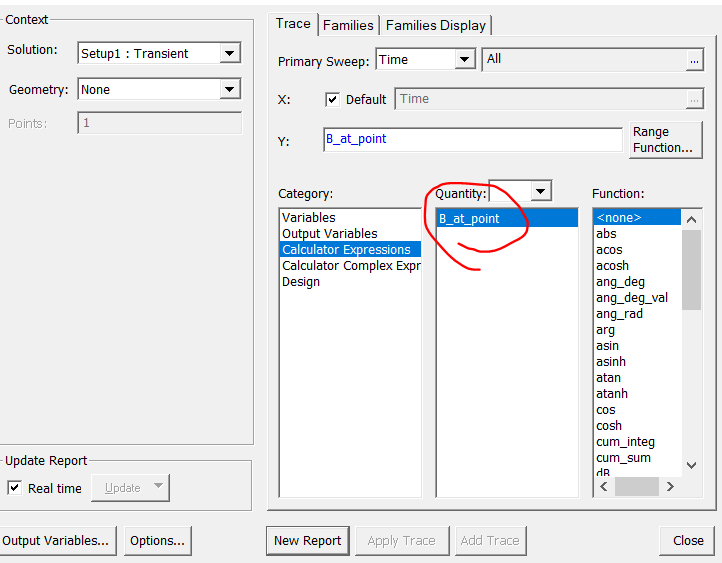## Electronics

•enathan1
Subscriber

I am trying to use the ANSYS Maxwell3D Transient to simulate the field produced by some large coils. I have applied a time varying current excitation to the coils. However, I cannot find a way to show the B-Field strength with respect to time in the results. The best I can do is show an animation of a field overlay but I cannot get a table or graph showing field magnitude with respect to time. Is there a way to do this?

•Navya C
Ansys Employee

Hi,

If I understand it correctly, you want to plot the magnetic field quantity variation at a particular point around your coil or within the system.

To achieve this - create a small volume (using the braw > box ) as nonmodel at the point where you want to find the field quantity.

Now using the maxwell filed calculator create an expression for the average field per unit volume of that non-model box.

now you can plot that calculator expression, which gives you the filed at that point with respect to time.

Regards

Navya

•enathan1
Subscriber

Navya,

Thank you for the help! Yes you understand this correctly; I would like a curve/data for the field at this point wrt time.

Do you mean that I can plot this in results, say as a "rectangular plot" or "data table"? Because when I try to do this there seems to be no place I can select my newly created calculator expression.

•Navya C
Ansys Employee

Hi,

Yes, you can plot as a rectangular plot.

Once you got your equations formed correctly in Maxwell Calculator > Click on Add > Give an appropriate name on the popup window

Then it will add the equation with that name in the Named expressions above.

Now you can RMC Results > Create Field Report > Rectangular plot > Calculator expressions > selecct your newly created expression

In the below image example I named my expression as "B_at_Point"

Mark this response as "is answer" if it answers your question for the benefit  of other studentsRegards

Navya

•engin
Subscriber
Hi Nchode, I have read your comment thanks it seems useful, but what type of calculation we can write on the field calculator. Can you write this formula order please.